Revisited: How Many Blocks are in that Model?

A long time ago, Seth wrote a post showing how to count the number of blocks in a model, including referenced models. At that time, using the functions find_mdlrefs to find all the referenced models and running sldiagnostics on each of them was the way to go.

In R2017b, Simulink Check introduces a new and easy way of finding out how many blocks are in a Simulink models, along with a lot of associated metrics: The Metrics Dashboard.

Block Count

To understand the sort of data that the Dashboard Metrics includes, I think it is a good idea to start with a simple model. For that, I will use the example model sf_car.slx. To get started, I launch the Dashboard Metrics from the Analysis menu: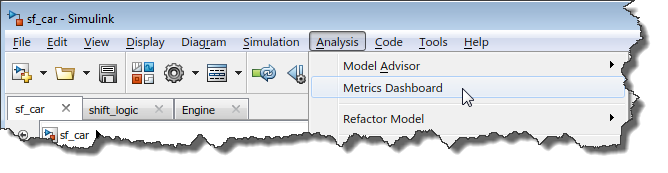Then I click the All Metrics button to begin the analysis: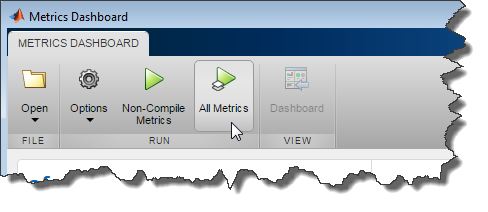Within the few seconds, I get the following report: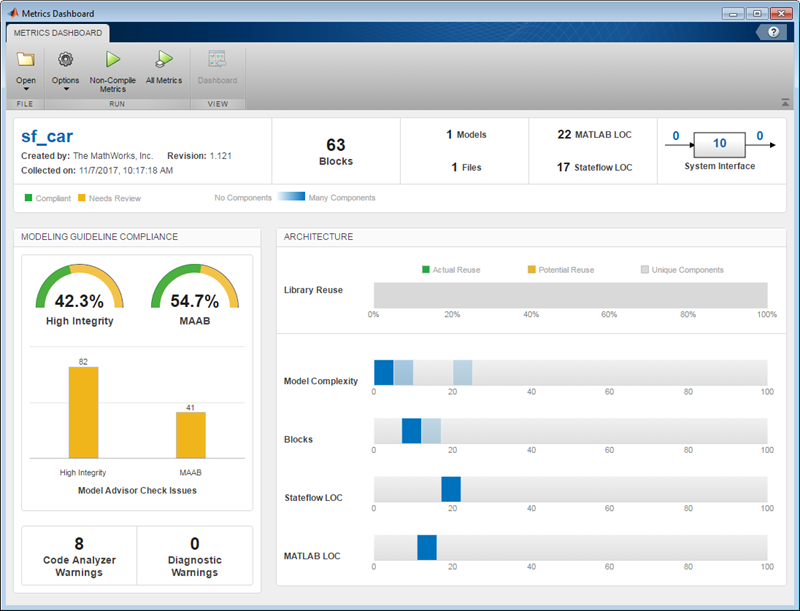Let's click on some of those metrics to see what they can tell us. To begin, let's click on the number of blocks. This opens a table listing all the components in the model, and how many blocks they contain.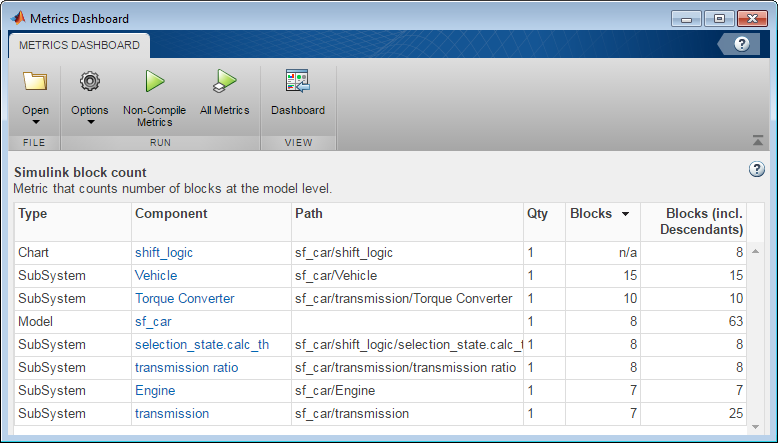For example, we can see that the Transmission Subsystem contains 7 blocks, and 25 blocks including its descendants: Torque Converter (10 blocks) and Transmission Ratio (8 blocks).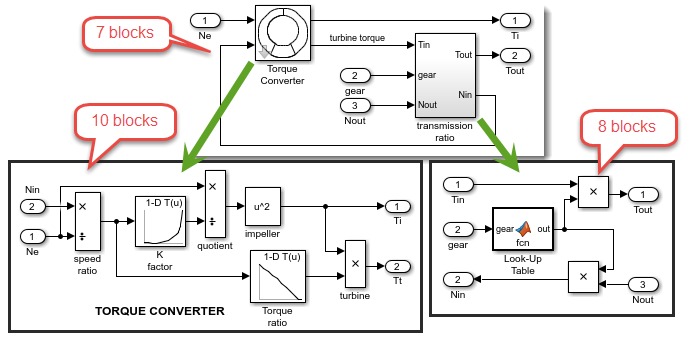More Metrics

In a way similar to the block count, the Metrics Dashboard can tell you how many and where in your model are the lines of MATLAB code (inside MATLAB Function blocks), lines of code inside Stateflow charts, and parameters.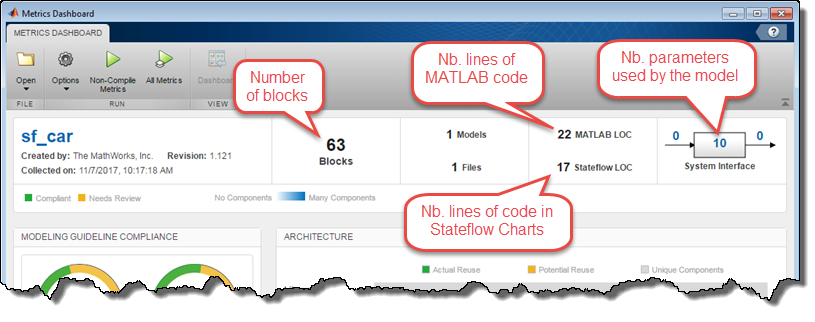In the above image, if I click on the number 10 in the System Interface section, I can see that the Engine subsystem uses 4 parameters from the base workspace: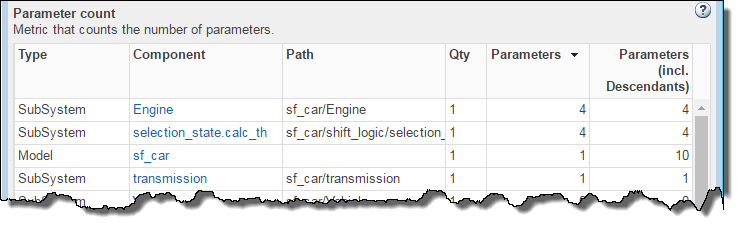After verification, I confirm that the Engine subsystem really uses 4 parameters: 3 in the Engine Torque Lookup Table, and 1 in the Gain block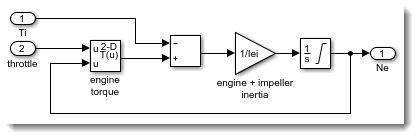Improving your model based on metrics

Now let's switch to a larger, more complex model. For that, I will use a hybrid electric vehicle example from the Powertrain Blockset.

One interesting metric for this model is the library reuse, which shows that many subsystems have identical copies in the model.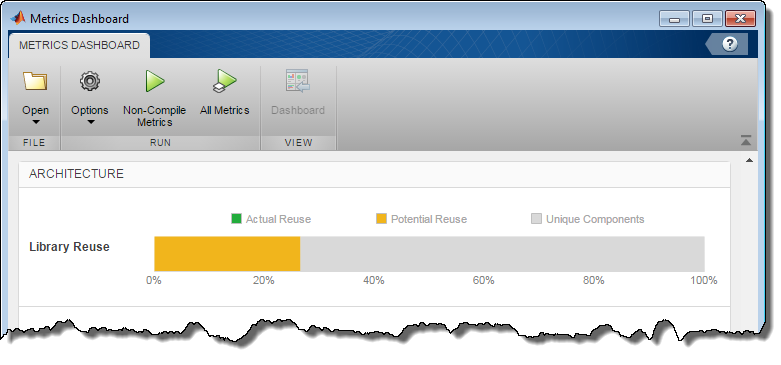If you click on the metric to look at the details, you will notice a button saying Open Conversion Tool: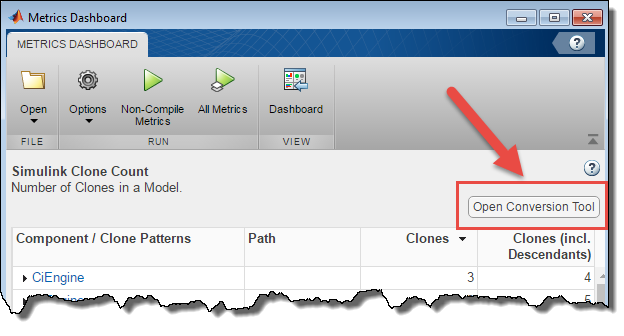This will launch a tool that can in one click replace all the copies of the subsystem in your model by instances of one library block.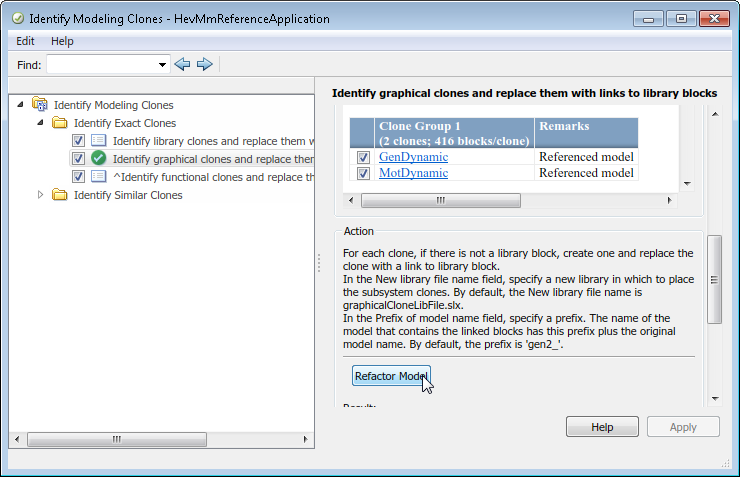As you can imagine, doing this refactoring should help to avoid situations where you could modify one copy of the subsystem and forget to modify the other copies.